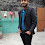## Restaurant Customers cses problem solution -

### Problem Statement-

• Time limit: 1.00 s
•
• Memory limit: 512 MB
You are given the arrival and leaving times of $n$ customers in a restaurant.

What was the maximum number of customers?

Input

The first input line has an integer $n$: the number of customers.

After this, there are $n$ lines that describe the customers. Each line has two integers $a$ and $b$: the arrival and leaving times of a customer.

You may assume that all arrival and leaving times are distinct.

Output

Print one integer: the maximum number of customers.

Constraints
• $1\le n\le 2\cdot {10}^{5}$
• $1\le a
Example

Input:
35 82 43 9

Output:
2

### solution-

Step 1- take input in a  vector which contain pair of int and bool and insert arrival time as true and leaving time as false.
Step 2- sort the vector.
stem 3- iterate over vector and when you found true update max if max > ans
step 4- else if you strike false update ans as ans-1

Here is code solution -

1.can u give me soulution

1.#include
using namespace std;
#define int long long int
int32_t main(){
ios::sync_with_stdio(false);
cin.tie(NULL);
int n;
cin>>n;
vector> v;
int x; int y;
while(n--){
cin>>x;cin>>y;
v.push_back(make_pair(x,true));
v.push_back(make_pair(y,false));
}
sort(v.begin(),v.end());
int ans =0; int maxx =0;
for(int i=0;i<v.size();i++){
if(v[i].second==true){
ans++;
maxx = max(ans,maxx);
}else{
ans--;
}
}
cout<<maxx<<endl;
return 0;
}